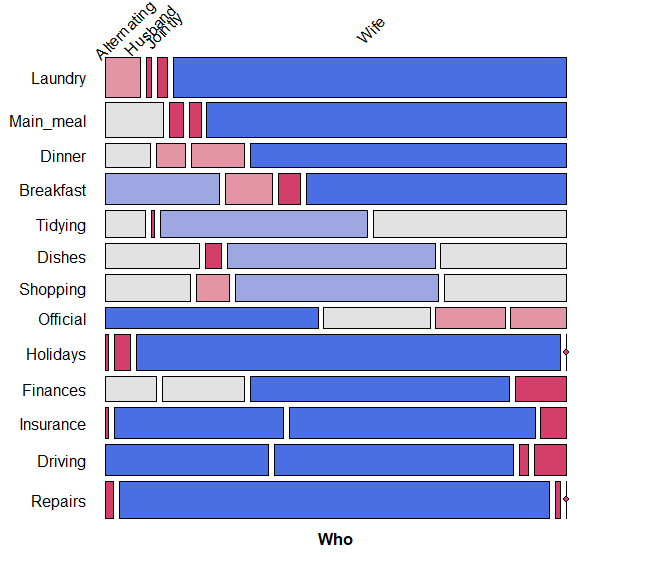# Mosaic plots

#### 2023-08-21

Mosaic plots provide an ideal method both for visualizing contingency tables and for visualizing the fit— or more importantly— lack of fit of a loglinear model.

For a two-way table, mosaic(), by default, fits a model of independence, $$[A][B]$$ or ~A + B as an R formula. The vcdExtra package extends this to models fit using glm(..., family=poisson), which can include specialized models for ordered factors, or square tables that are intermediate between the saturated model, $$[A B]$$ = A * B, and the independence model $$[A][B]$$.

For $$n$$-way tables, vcd::mosaic() can fit any loglinear model, and can also be used to plot a model fit with MASS:loglm(). The vcdExtra package extends this to models fit using stats::glm() and, by extension, to non-linear models fit using the gnm package.

See Friendly (1994), Friendly (1999) for the statistical ideas behind these uses of mosaic displays in connection with loglinear models. Our book Friendly & Meyer (2016) gives a detailed discussion of mosaic plots and many more examples.

The essential ideas are to:

• recursively sub-divide a unit square into rectangular “tiles” for the cells of the table, such that the area of each tile is proportional to the cell frequency. Tiles are split in a sequential order:

• First according to the marginal proportions of a first variable, V1
• Next according to the conditional proportions of a 2nd variable, V2 | V1
• Next according to the conditional proportions of a 3rd variable, V3 | {V1, V2}
• For a given loglinear model, the tiles can then be shaded in various ways to reflect the residuals (lack of fit) for a given model.

• The pattern of residuals can then be used to suggest a better model or understand where a given model fits or does not fit.

mosaic() provides a wide range of options for the directions of splitting, the specification of shading, labeling, spacing, legend and many other details. It is actually implemented as a special case of a more general class of displays for $$n$$-way tables called strucplot, including sieve diagrams, association plots, double-decker plots as well as mosaic plots.

For details, see help(strucplot) and the “See also” links therein, and also Meyer, Zeileis, & Hornik (2006), which is available as an R vignette via vignette("strucplot", package="vcd").

Example: A mosaic plot for the Arthritis treatment data fits the model of independence, ~ Treatment + Improved and displays the association in the pattern of residual shading. The goal is to visualize the difference in the proportions of Improved for the two levels of Treatment : “Placebo” and “Treated”.

The plot below is produced with the following call to mosaic(). With the first split by Treatment and the shading used, it is easy to see that more people given the placebo experienced no improvement, while more people given the active treatment reported marked improvement.

data(Arthritis, package="vcd")
art <- xtabs(~Treatment + Improved, data = Arthritis)
split_vertical = TRUE,
main="Arthritis: [Treatment] [Improved]")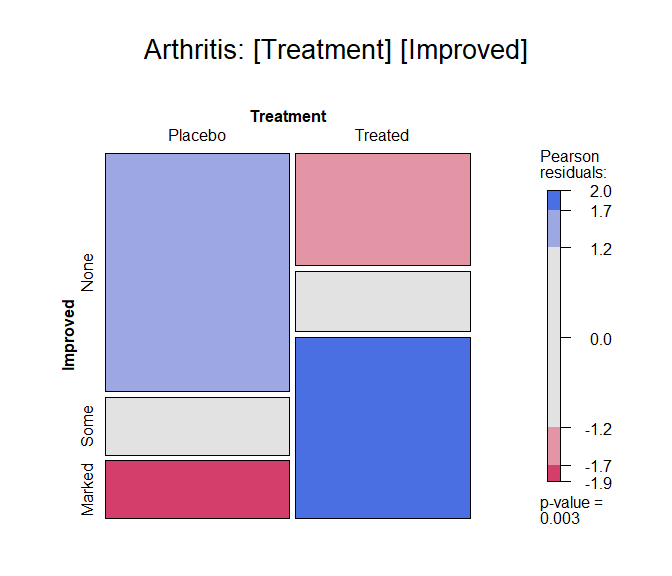Mosaic plot for the Arthritis data, using shading_max

gp = shading_max specifies that color in the plot signals a significant residual at a 90% or 99% significance level, with the more intense shade for 99%. Note that the residuals for the independence model were not large (as shown in the legend), yet the association between Treatment and Improved is highly significant.

summary(art)
## Call: xtabs(formula = ~Treatment + Improved, data = Arthritis)
## Number of cases in table: 84
## Number of factors: 2
## Test for independence of all factors:
##  Chisq = 13.055, df = 2, p-value = 0.001463

In contrast, one of the other shading schemes, from Friendly (1994) (use: gp = shading_Friendly), uses fixed cutoffs of $$\pm 2, \pm 4$$, to shade cells which are individually significant at approximately $$\alpha = 0.05$$ and $$\alpha = 0.001$$ levels, respectively. The plot below uses gp = shading_Friendly.

mosaic(art, gp = shading_Friendly,
split_vertical = TRUE,
main="Arthritis: gp = shading_Friendly")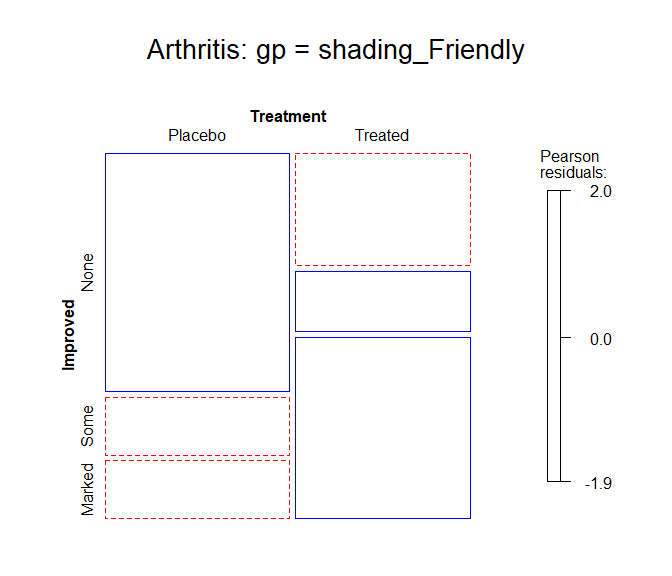Mosaic plot for the Arthritis data, using shading_Friendly

## Permuting variable levels

Mosaic plots using tables or frequency data frames as input typically take the levels of the table variables in the order presented in the dataset. For character variables, this is often alphabetical order. That might be helpful for looking up a value, but is unhelpful for seeing and understanding the pattern of association.

It is usually much better to order the levels of the row and column variables to help reveal the nature of their association. This is an example of effect ordering for data display (Friendly & Kwan, 2003).

Example:

Data from Glass (1954) gave this 5 x 5 table on the occupations of 3500 British fathers and their sons, where the occupational categories are listed in alphabetic order.

data(Glass, package="vcdExtra")
(glass.tab <- xtabs(Freq ~ father + son, data=Glass))
##               son
## father         Managerial Professional Skilled Supervisory Unskilled
##   Managerial          174           28     154          84        55
##   Professional         45           50      18           8         8
##   Skilled             150           14     714         185       447
##   Supervisory          78           11     223         110        96
##   Unskilled            42            3     320          72       411

The mosaic display shows very strong association, but aside from the diagonal cells, the pattern is unclear. Note the use of set_varnames to give more descriptive labels for the variables and abbreviate the occupational category labels. and interpolate to set the shading levels for the mosaic.

largs <- list(set_varnames=list(father="Father's Occupation",
son="Son's Occupation"),
abbreviate=10)
gargs <- list(interpolate=c(1,2,4,8))

mosaic(glass.tab,
labeling_args=largs,
gp_args=gargs,
main="Alphabetic order",
legend=FALSE,
rot_labels=c(20,90,0,70))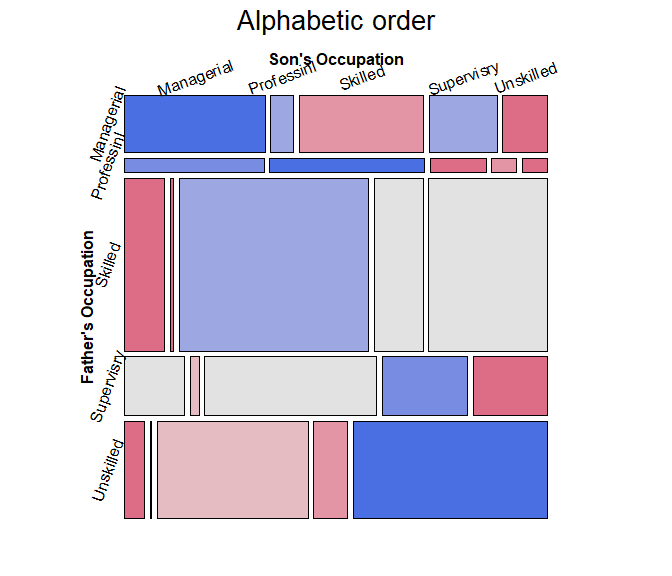The occupational categories differ in status, and can be reordered correctly as follows, from Professional down to Unskilled.

# reorder by status
ord <- c(2, 1, 4, 3, 5)
row.names(glass.tab)[ord]
##  "Professional" "Managerial"   "Supervisory"  "Skilled"      "Unskilled"

The revised mosaic plot can be produced by indexing the rows and columns of the table using ord.

mosaic(glass.tab[ord, ord],
labeling_args=largs,
gp_args=gargs,
main="Effect order",
legend=FALSE,
rot_labels=c(20,90,0,70))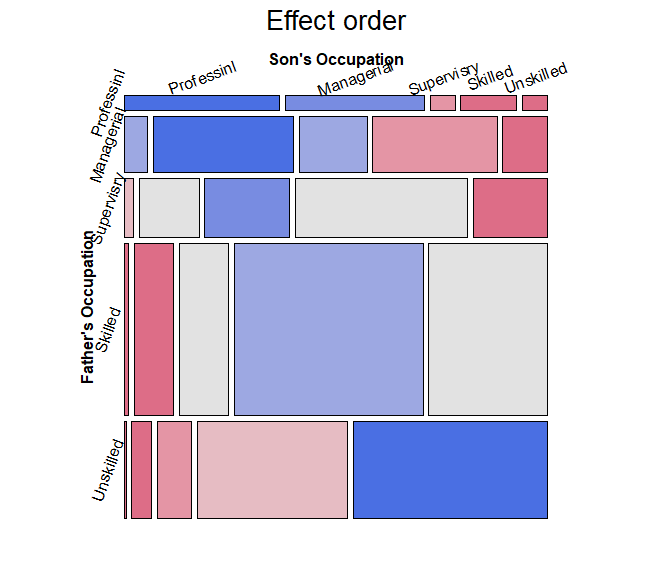From this, and for the examples in the next section, it is useful to re-define father and son as ordered factors in the original Glass frequency data.frame.

Glass.ord <- Glass
Glass.ord$father <- ordered(Glass.ord$father, levels=levels(Glass$father)[ord]) Glass.ord$son    <- ordered(Glass.ord$son, levels=levels(Glass$son)[ord])
str(Glass.ord)
## 'data.frame':    25 obs. of  3 variables:
##  $father: Ord.factor w/ 5 levels "Professional"<..: 1 1 1 1 1 2 2 2 2 2 ... ##$ son   : Ord.factor w/ 5 levels "Professional"<..: 1 2 3 4 5 1 2 3 4 5 ...
##  $Freq : int 50 45 8 18 8 28 174 84 154 55 ... ## Square tables For mobility tables such as this, where the rows and columns refer to the same occupational categories it comes as no surprise that there is a strong association in the diagonal cells: most often, sons remain in the same occupational categories as their fathers. However, the re-ordered mosaic display also reveals something subtler: when a son differs in occupation from the father, it is more likely that he will appear in a category one-step removed than more steps removed. The residuals seem to decrease with the number of steps from the diagonal. For such tables, specialized loglinear models provide interesting cases intermediate between the independence model, [A] [B], and the saturated model, [A B]. These can be fit using glm(), with the data in frequency form, glm(Freq ~ A + B + assoc, data = ..., family = poisson) where assoc is a special term to handle a restricted form of association, different from A:B which specifies the saturated model in this notation. • Quasi-independence: Asserts independence, but ignores the diagonal cells by fitting them exactly. The loglinear model is: $$\log m_{ij} = \mu + \lambda^A_i + \lambda^B_j + \delta_i I(i = j)$$, where $$I()$$ is the indicator function. • Symmetry: This model asserts that the joint distribution of the row and column variables is symmetric, that is $$\pi_{ij} = \pi_{ji}$$: A son is equally likely to move from their father’s occupational category $$i$$ to another category, $$j$$, as the reverse, moving from $$j$$ to $$i$$. Symmetry is quite strong, because it also implies marginal homogeneity, that the marginal probabilities of the row and column variables are equal, $$\pi{i+} = \sum_j \pi_{ij} = \sum_j \pi_{ji} = \pi_{+i}$$ for all $$i$$. • Quasi-symmetry: This model uses the standard main-effect terms in the loglinear model, but asserts that the association parameters are symmetric, $$\log m_{ij} = \mu + \lambda^A_i + \lambda^B_j + \lambda^{AB}_{ij}$$, where $$\lambda^{AB}_{ij} = \lambda^{AB}_{ji}$$. The gnm package provides a variety of these functions: gnm::Diag(), gnm::Symm() and gnm::Topo() for an interaction factor as specified by an array of levels, which may be arbitrarily structured. For example, the following generates a term for a diagonal factor in a $$4 \times 4$$ table. The diagonal values reflect parameters fitted for each diagonal cell. Off-diagonal values, “.” are ignored. rowfac <- gl(4, 4, 16) colfac <- gl(4, 1, 16) diag4by4 <- Diag(rowfac, colfac) matrix(Diag(rowfac, colfac, binary = FALSE), 4, 4) ## [,1] [,2] [,3] [,4] ## [1,] "1" "." "." "." ## [2,] "." "2" "." "." ## [3,] "." "." "3" "." ## [4,] "." "." "." "4" Symm() constructs parameters for symmetric cells. The particular values don’t matter. All that does matter is that the same value, e.g., 1:2 appears in both the (1,2) and (2,1) cells. symm4by4 <- Symm(rowfac, colfac) matrix(symm4by4, 4, 4) ## [,1] [,2] [,3] [,4] ## [1,] "1:1" "1:2" "1:3" "1:4" ## [2,] "1:2" "2:2" "2:3" "2:4" ## [3,] "1:3" "2:3" "3:3" "3:4" ## [4,] "1:4" "2:4" "3:4" "4:4" Example: To illustrate, we fit the four models below, starting with the independence model Freq ~ father + son and then adding terms to reflect the restricted forms of association, e.g., Diag(father, son) for diagonal terms and Symm(father, son) for symmetry. library(gnm) glass.indep <- glm(Freq ~ father + son, data = Glass.ord, family=poisson) glass.quasi <- glm(Freq ~ father + son + Diag(father, son), data = Glass.ord, family=poisson) glass.symm <- glm(Freq ~ Symm(father, son), data = Glass.ord, family=poisson) glass.qsymm <- glm(Freq ~ father + son + Symm(father, son), data = Glass.ord, family=poisson) We can visualize these using the vcdExtra::mosaic.glm() method, which extends mosaic displays to handle fitted glm objects. Technical note: for models fitted using glm(), standardized residuals, residuals_type="rstandard" have better statistical properties than the default Pearson residuals in mosaic plots and analysis. mosaic(glass.quasi, residuals_type="rstandard", shade=TRUE, labeling_args=largs, gp_args=gargs, main="Quasi-Independence", legend=FALSE, rot_labels=c(20,90,0,70) )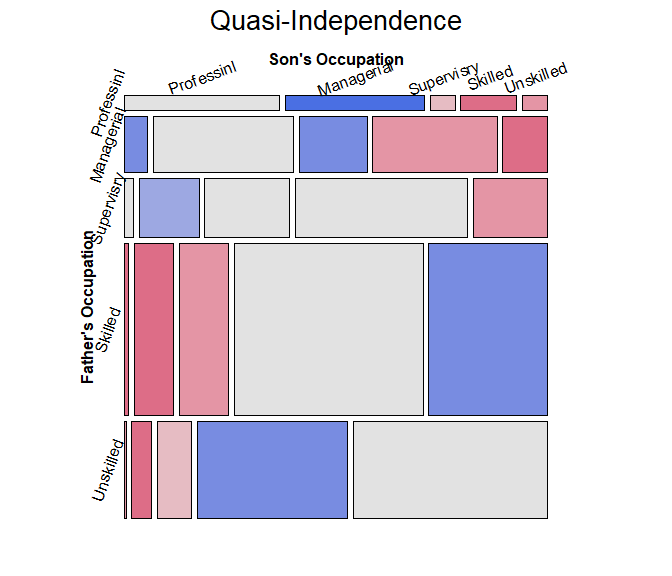Mosaic plots for the other models would give further visual assessment of these models, however we can also test differences among them. For nested models, anova() gives tests of how much better a more complex model is compared to the previous one. # model comparisons: for *nested* models anova(glass.indep, glass.quasi, glass.qsymm, test="Chisq") ## Analysis of Deviance Table ## ## Model 1: Freq ~ father + son ## Model 2: Freq ~ father + son + Diag(father, son) ## Model 3: Freq ~ father + son + Symm(father, son) ## Resid. Df Resid. Dev Df Deviance Pr(>Chi) ## 1 16 792.19 ## 2 11 235.78 5 556.41 < 2.2e-16 *** ## 3 6 4.66 5 231.12 < 2.2e-16 *** ## --- ## Signif. codes: 0 '***' 0.001 '**' 0.01 '*' 0.05 '.' 0.1 ' ' 1 Alternatively, vcdExtra::LRstats() gives model summaries for a collection of models, not necessarily nested, with AIC and BIC statistics reflecting model parsimony. models <- glmlist(glass.indep, glass.quasi, glass.symm, glass.qsymm) LRstats(models) ## Likelihood summary table: ## AIC BIC LR Chisq Df Pr(>Chisq) ## glass.indep 960.91 971.88 792.19 16 < 2.2e-16 *** ## glass.quasi 414.50 431.57 235.78 11 < 2.2e-16 *** ## glass.symm 218.18 236.47 37.46 10 4.704e-05 *** ## glass.qsymm 193.38 216.54 4.66 6 0.5876 ## --- ## Signif. codes: 0 '***' 0.001 '**' 0.01 '*' 0.05 '.' 0.1 ' ' 1 By all criteria, the model of quasi symmetry fits best. The residual deviance$G^2 is not significant. The mosaic is largely unshaded, indicating a good fit, but there are a few shaded cells that indicate the remaining positive and negative residuals. For comparative mosaic displays, it is sometimes useful to show the $$G^2$$ statistic in the main title, using vcdExtra::modFit() for this purpose.

mosaic(glass.qsymm,
residuals_type="rstandard",
labeling_args=largs,
gp_args=gargs,
main = paste("Quasi-Symmetry", modFit(glass.qsymm)),
legend=FALSE,
rot_labels=c(20,90,0,70)
)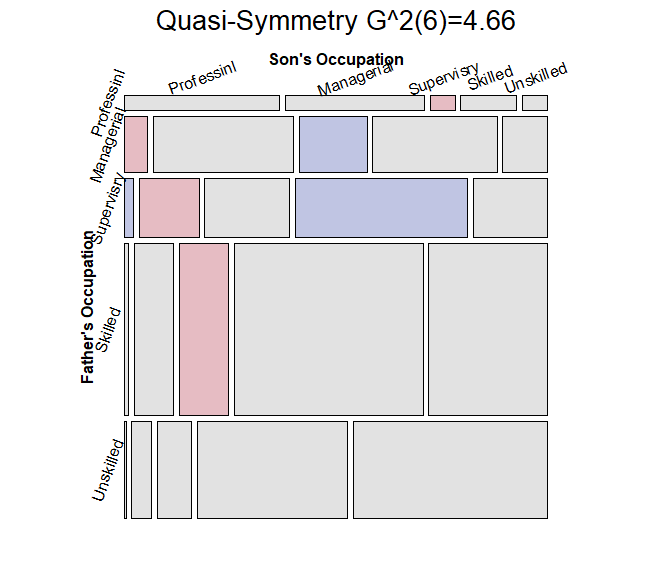## Correspondence analysis ordering

When natural orders for row and column levels are not given a priori, we can find orderings that make more sense using correspondence analysis.

The general ideas are that:

• Correspondence analysis assigns scores to the row and column variables to best account for the association in 1, 2, … dimensions

• The first CA dimension accounts for largest proportion of the Pearson $$\chi^2$$

• Therefore, permuting the levels of the row and column variables by the CA Dim1 scores gives a more coherent mosaic plot, more clearly showing the nature of the association.

• The seriation package now has a method to order variables in frequency tables using CA.

Example: As an example, consider the HouseTasks dataset, a 13 x 4 table of frequencies of household tasks performed by couples, either by the Husband, Wife, Alternating or Jointly. You can see from the table that some tasks (Repairs) are done largely by the husband; some (laundry, main meal) are largely done by the wife, while others are done jointly or alternating between husband and wife. But the Task and Who levels are both in alphabetical order.

data("HouseTasks", package = "vcdExtra")
##            Who
## Task        Alternating Husband Jointly Wife
##   Breakfast          36      15       7   82
##   Dinner             11       7      13   77
##   Dishes             24       4      53   32
##   Driving            51      75       3   10
##   Finances           13      21      66   13
##   Holidays            1       6     153    0
##   Insurance           1      53      77    8
##   Laundry            14       2       4  156
##   Main_meal          20       5       4  124
##   Official           46      23      15   12
##   Repairs             3     160       2    0
##   Shopping           23       9      55   33
##   Tidying            11       1      57   53

The naive mosaic plot for this dataset is shown below, splitting first by Task and then by Who. Due to the length of the factor labels, some features of labeling were used to make the display more readable.

require(vcd)
labeling = labeling_border(rot_labels = c(45,0, 0, 0),
offset_label =c(.5,5,0, 0),
varnames = c(FALSE, TRUE),
just_labels=c("center","right"),
tl_varnames = FALSE),
legend = FALSE)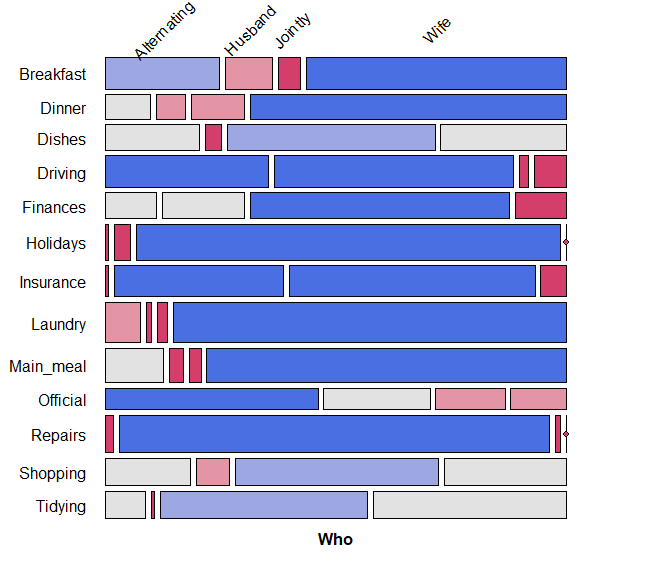Correspondence analysis, using the ca package, shows that nearly 89% of the $$\chi^2$$ can be accounted for in two dimensions.

require(ca)
summary(HT.ca, rows=FALSE, columns=FALSE)
##
## Principal inertias (eigenvalues):
##
##  dim    value      %   cum%   scree plot
##  1      0.542889  48.7  48.7  ************
##  2      0.445003  39.9  88.6  **********
##  3      0.127048  11.4 100.0  ***
##         -------- -----
##  Total: 1.114940 100.0

The CA plot has a fairly simple interpretation: Dim1 is largely the distinction between tasks primarily done by the wife vs. the husband. Dim2 distinguishes tasks that are done singly vs. those that are done jointly.

plot(HT.ca, lines = TRUE)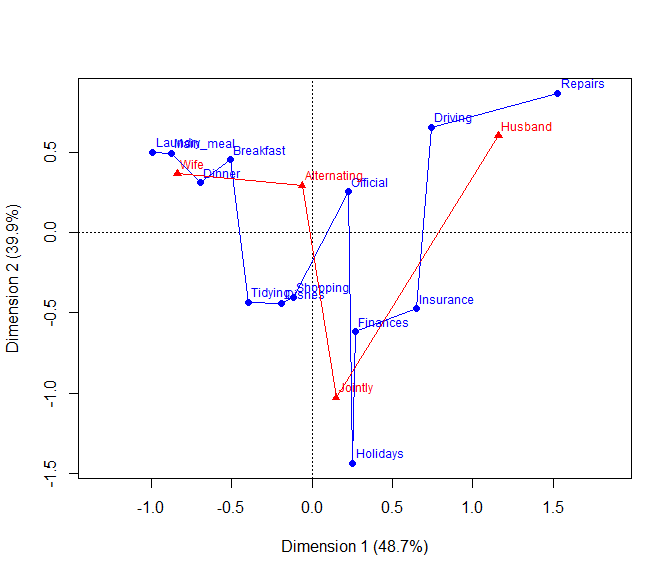So, we can use the CA method of seriation::seriate() to find the order of permutations of Task and Who along the CA dimensions.

require(seriation)
order <- seriate(HouseTasks, method = "CA")
# the permuted row and column labels
##   "Laundry"   "Main_meal" "Dinner"    "Breakfast" "Tidying"   "Dishes"
##   "Shopping"  "Official"  "Holidays"  "Finances"  "Insurance" "Driving"
##  "Repairs"
##  "Wife"        "Alternating" "Jointly"     "Husband"

Now, use seriation::permute() to use order for the permutations of Task and Who, and plot the resulting mosaic:

# do the permutation
legend = FALSE)